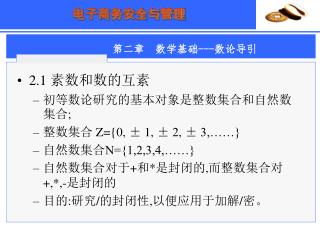# 2.1 素数和数的互素 初等数论研究的基本对象是整数集合和自然数集合 ; 整数集合 Z={0, ± 1, ± 2, ± 3,……} 自然数集合 N={1,2,3,4,……} - PowerPoint PPT PresentationDownload Presentation2.1 素数和数的互素 初等数论研究的基本对象是整数集合和自然数集合 ; 整数集合 Z={0, ± 1, ± 2, ± 3,……} 自然数集合 N={1,2,3,4,……}

2.1 素数和数的互素 初等数论研究的基本对象是整数集合和自然数集合 ; 整数集合 Z={0, ± 1, ± 2, ± 3,……} 自然数集合 N={1,2,3,4,……}
Download Presentation## 2.1 素数和数的互素 初等数论研究的基本对象是整数集合和自然数集合 ; 整数集合 Z={0, ± 1, ± 2, ± 3,……} 自然数集合 N={1,2,3,4,……}

- - - - - - - - - - - - - - - - - - - - - - - - - - - E N D - - - - - - - - - - - - - - - - - - - - - - - - - - -
##### Presentation Transcript

1. 2.1 素数和数的互素 初等数论研究的基本对象是整数集合和自然数集合; 整数集合 Z={0, ± 1, ± 2, ± 3,……} 自然数集合N={1,2,3,4,……} 自然数集合对于+和*是封闭的,而整数集合对+,*,-是封闭的 目的:研究/的封闭性,以便应用于加解/密。

2. 2.1 素数和数的互素 2.1.1 除数和整除的概念 若b≠0且a,b,m∈Z，使得a=mb，称b整除a,记为b|a，又称b为a的除数（因子）。若不存在整数m使得a=mb，则称b不能整除a。 例：24的因子是1，2，3，4，6，8，12，24 几个性质： 如果a|1，则a= ±1; 如果a|b且b|a，则a= ±b; 任何b ≠0能整除0； 如果b|g,而且b|h，则对任意整数m和n，有b|(mg+nh)

3. 2.1 素数和数的互素 2.1.2 素数（质数）的概念 整数p>1被称为素数，是指p的因子仅有±1， ±p 算术基本定理 任何大于1的整数a，都能被因式分解为：a=p1a1p2a2…ptat，这里p1>p2>…>pt都是素数，且每一个ai>0。 例：91=13×7；11011=13×112×7；12=3×22

4. 2.1 素数和数的互素 2.1.3 最大公约数 若a,b,c∈Z，如果c|a,c|b,称c是a和b的公约数。 正整数d称为a和b的最大公约数，如果它满足：1）d是a和b的公约数；2）对a和b的任何一个公约数c,有c|d，记为：d=gcd(a,b)。注意： 第2)指出了其它约数都是最大公约数的因子，这一点可以从: a=p1a1p2a2…ptat， , b=q1b1q2b2…qfbf , c=rimin(ai,bi)…rjmin(aj,bj)看出(这里ri=pm=qn)。例如gcd(8,12)=4,其它约数2,1都是4的因子。 最大公约数的另外一种等价形式的定义：gcd(a,b)=max{k| k|a,k|b}

5. 2.1 素数和数的互素 2.1.4 带余除法 命题：设a,b ∈Z,b>0，则存在惟一确定的整数q和r，使得a=qb+r （0≤r<b)。例如7=2 ×3+1；-7=-3 ×3+2 证明： 定义实数x=[x]+{x}，其中[x]为x的整数部分，{x}为x的小数部分； 先证明满足条件的q和r是存在的：令q=[a/b],r=a-qb,则q和r都是整数；并且由于r/b=a/b-q={a/b},而0≤{a/b}<1,因而0 ≤r/b<1,即0 ≤r<b。 再证明q和r 是惟一确定的：如果又有整数q’和r’使得a=q’b+r’(0 ≤r’<b),则有0 ≤|r-r’|<b且r-r’=b(q’-q)，即q-q’=0或q=q’,从而r=r’。

6. 2.1 素数和数的互素 2.1.5 欧几里德算法Euclid（辗转相除法，求最大公约数的方法） a=q1b+r1=q1(q2r1+r2)+r1=…=q1(q2(…(qnrn-1+0)+…)+r1,直到rn=0为止，则gcd(a,b)=rn-1 计算机编程的方法： (1) X←a; Y←b; (2)if Y=0 return X=gcd(a,b); (3)R=X mod Y; (4)X←Y,Y←R; (5) goto (2);

7. 2.1 素数和数的互素 2.1.5 欧几里德算法Euclid（辗转相除法，求最大公约数的方法） 例：求gcd(1970,1066) 1970=1×1066+904 1066=1×904+162 … 6=1×4+2 4=2×2+0 所以：gcd(1970,1066)=gcd(4,2)=2

8. 2.1 素数和数的互素 2.1.5 欧几里德算法Euclid（辗转相除法，求最大公约数的方法） 引理：任意两个整数 a,b的最大公约数d可以表示成a,b两数关于整系数的线性组合，即有s,t ∈Z,使d=sa+tb。 例如gcd(180,252)=36, 252=1*180+72（72=1*252-1*180），180=2*72+36(36=1*180-2*72),72=2*36+0 gcd(180,252)=1*180-2*72=1*180-2*(1*252-1*180)=3*180-2*252 数的互素：若gcd(a,b)=1，则称a与b互素（ a,b ∈ Z）

9. 2.2 模运算 2.2.1 整数同余 定义：如果(a mod n)=(b mod n)，则称整数a和b模n同余，记为a≡b mod n，这里n为正整数。 整数同余关系是一种等价关系，满足三个性质： 自反性 对称性 传递性

10. 2.2 模运算 2.2.2 模运算的操作与性质 [(a mod n) ±×(b mod n)] mod n=(a±×b) mod n 例：求117 mod 13

11. 2.2 模运算 2.2.3 幺元e（单位元）和零元θ 若e⊙x ≡x ⊙e ≡x (mod n),则称e为⊙模运算的幺元；例如，0是Z集合中+或-模运算的幺元。 若θ⊙x ≡x ⊙ θ≡ θ(mod n),则称θ为⊙模运算的零元；例如，0是Z集合中*模运算的零元。

12. 2.2 模运算 2.2.4 可逆元（相反数） 若x⊙y ≡e (mod n),则称x(y)可逆，其逆元为y(x)。 例如： 1+7 ≡0(mod 8),则1的逆元为7，记为1-1=7 (mod 8); 3*3 ≡1(mod 8),则3的逆元为3，记为3-1=3 (mod 8); 在此基础上可定义除法运算：3/3=3*3-1=3*3 ≡1 (mod 8) 注意：集合{0，1，2，3，4，5，6，7}上的*运算，并不是每个元素都有逆元。

13. 2.2 模运算 2.2.5 整数环和剩余类环 Z是整数环，其运算+、*满足封闭性、交换律、结合律和分配律； 整数环Z模正整数n得到的剩余类集合Zn( 或Z/(n))称为剩余类环，即Zn={,,,…,[n-1]} 在剩余类环中，存在两类元素： 零因子：若α,β ∈Zn且α,β ≠，有α*β≡ (mod n) 可逆元因子：若α,β ∈Zn，有α*β≡ (mod n) 例如：Z8的零因子有：，，，，可逆元因子有：，，， 问题：可逆元因子有何特征？其个数有多少？

14. 2.2 模运算 可逆元因子特征定律： 剩余类环Zn中元素a=[a]为可逆元因子当且仅当gcd(a,n)=1(即a与n互素) 证明： (1)充分性： gcd(a,n)=1=> a为可逆元因子。由gcd(a,n)=1根据欧几里德引理有：1=sa+tn, 1 =(sa+tn) mod n, 1 ≡sa mod n,即a的逆元为s,a为可逆元因子； (2)必要性：a为可逆元因子=>gcd(a,n)=1。sa ≡1 mod n,即sa=tn+1,所以gcd(a,n)=1

15. 2.2 模运算 欧拉函数（Euler φ(n),可逆元因子的个数） 定义: 当n=1时，φ(1)=1; 当n>1时， φ(n)的值为Zn集合中与n互素的元素的个数 例如： φ(8)=4, φ(24)=8 若p 为素数，则φ(p)=p-1； 若p、q为不同的素数，则φ(pq)=(p-1)(q-1)=φ(p)φ(q) 若p 为素数，则φ(pp)=p(p-1)； 若ab≡ac(mod n)且gcd(a,n)=1，则b≡c (mod n)

16. 2.3 几个重要定理 2.3.1 费马定理（Format formula） 如果p为素数， a是任意一个正整数，a不能被p整除(此时gcd(a,p)=1)，则有ap-1≡1(mod p) 2.3.2 欧拉定理（Euler formula） 对任意互素的整数a和n，有a φ(n) ≡1(mod n) 注意： 费马定理是欧拉定理的特例； 在RSA 加密算法中，并没有要求整数a和n（n=pq,p和q为素数）互数，但也有a φ(n) ≡1(mod n)这样的结论成立。

17. 2.4 RSA算法原理 设p,q为大素数(n=pq, φ(n) =(p-1)(q-1)), a,b为满足ab ≡1(mod φ(n) )的两个整数，则： 公钥为：(n,b)，私钥为(p,q,a) 加密过程：对信息x(x<n)，密文y=xb mod n 解密过程：对密文 y, 还原明文x=ya mod n